Electron-positron Annihilation and Pair Creation.

Compton Scattering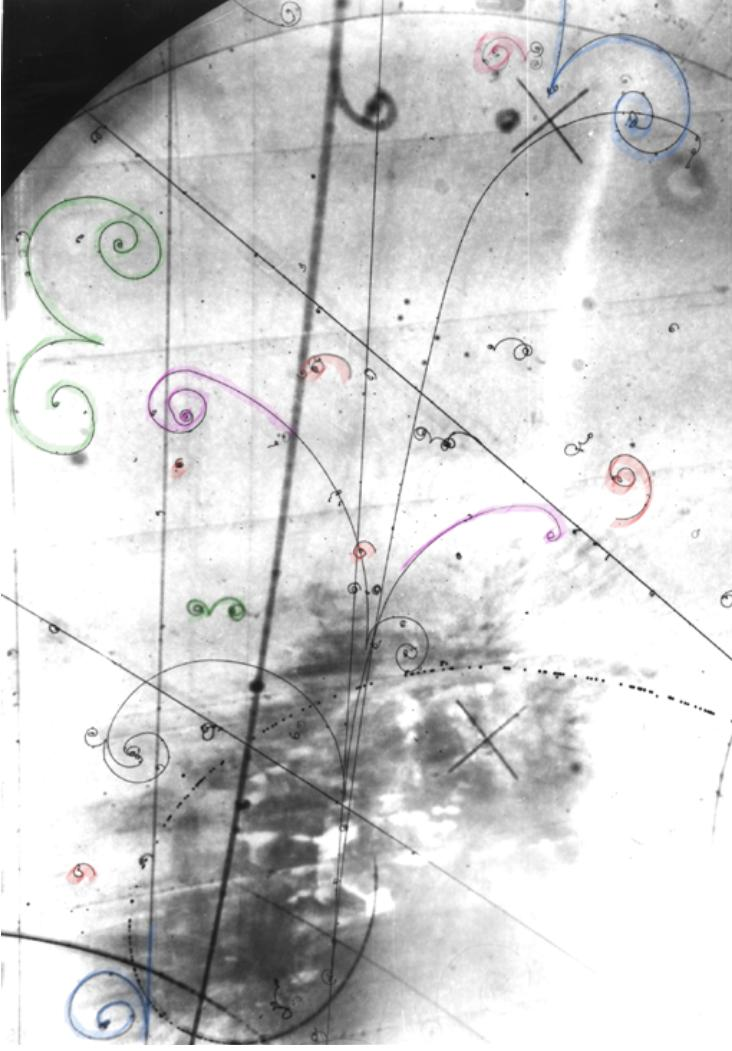Fig.1 This bubble chamber picture shows some electromagnetic events such as pair creation or materialization of high energy photon into an electron-positron pair (green tracks), the Compton effect (red tracks), the emission of electromagnetic radiation by accelerating charges (violet tracks) (bremsstrahlung) and the knock-on electrons or delta ray (blue tracks)

Electron-positron Annihilation

The processes of electro-positron annihilation into photons pairs and of pair creation by photon are of interest both theoretically and experimentally.

The electron’s antiparticle, the positron, is identical in mass but has a positive charge. If an electron and positron collide, they will annihilate with the production of two gamma photons: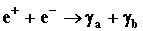Fig. 2  Part of a bubble-chamber picture from a neutrino experiment performed at the Fermilab (found at the University of Birmingham). A positron in flight annihilate with an electron. The photon that is produced materializes at a certain distance, along the line of flight, resulting a new electron-positron pair (marked with green)

In the frame of reference related to the electron, the conservation laws are: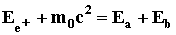(2.1)

where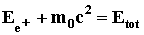the momentum conservation is: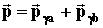(2.2)

The energy transferred to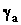is maximum whenis emitted in the direction of the incident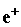.

Hence: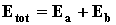(2.3)

and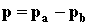(2.4)

but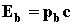and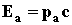because of the zero-masses of photons.

Multiplying (2.4) by c, gives: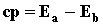(2.5)

Subtracting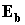from (2.5) and replacing it in (2.3) gives: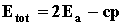(2.6)

Therefore: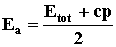(2.7)

where one can calculate the total energy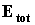and momentum of the incidentby using its kinetic energy T: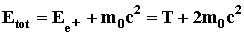(2.8)

and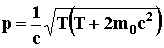(2.9)

Electron-Positron Pair Production

When a photon has quantum energy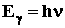higher than the rest energy of an electron plus a positron, one of the ways such as a photon interacts with matter is by producing an electron-positron pair in the field of nucleus.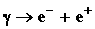This process cannot take place in free space because of the conservation laws violation.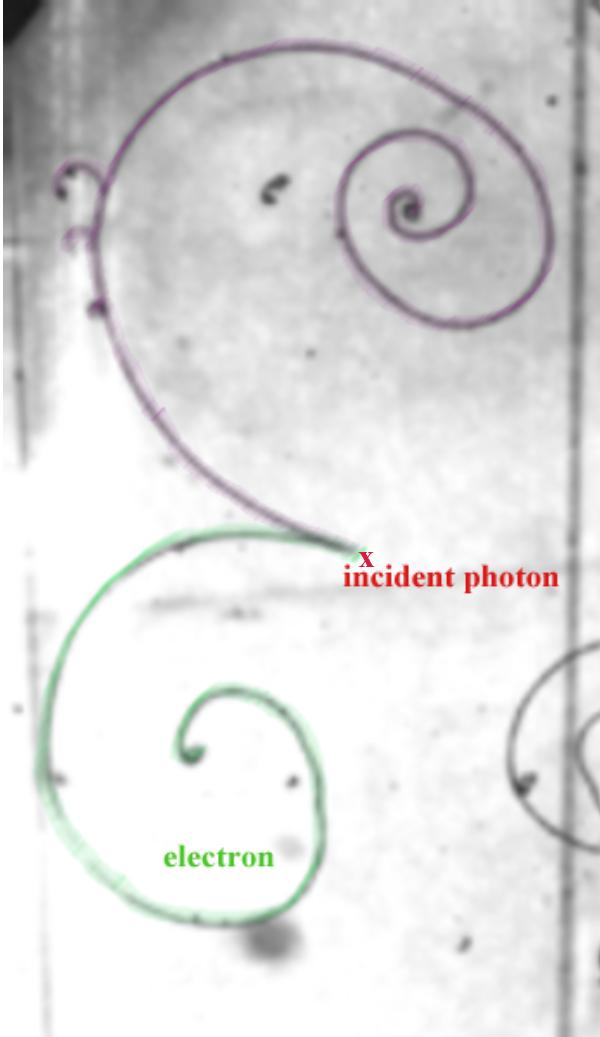Fig.3 Part of a bubble chamber picture (Fermilab'15 foot Bubble Chamber', found at the University of Birmingham). The curly line which turns to the left is an electron. Positron looks similar but turn to the right The magnetic field is perpendicular to the picture plan

The rest energy of the electron is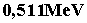, so for photons with energies much bigger than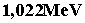, pair production is possible and can be a mode for the interaction of X-rays, or gamma-rays with matter.

Compton Scattering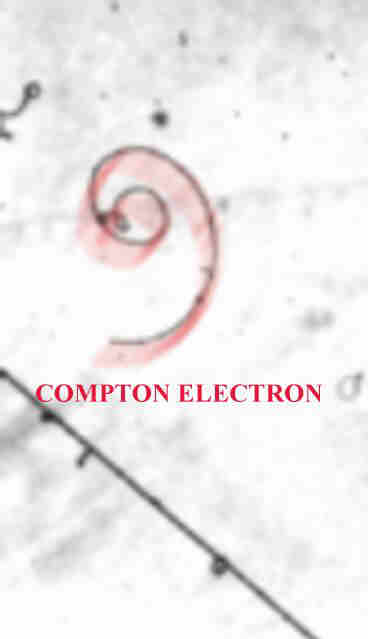Fig.3 Part of a bubble chamber picture (Fermilab'15 foot Bubble Chamber', found at the University of Birmingham). An electron was knocked out of an atom by a high energy photon.

Compton scattering is related by crossing symmetry to pair annihilation: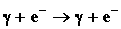Compton scattering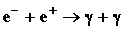Pair annihilation

It can be seen that the electron on the left side of the Compton process can be replaced by its antiparticle, the positron, on the other side of the interaction resulting pair annihilation.

Crossing symmetry applies to all known particles, including the photon, which is its own antiparticle.

Generally, in the case of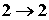processes, the crossing symmetry relates the following interactions: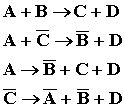The over bar indicates the antiparticle.

In the Compton effect one can observe a shift in wavelength upon scattering of light from stationary electron. The Compton effect, discovered by Compton in 1923, provided the final confirmation of the validity of Planck’s quantum hypothesis that electromagnetic radiation came in discrete mass less packets (photons) with energy proportional to frequency.

Let a photon of frequency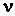or wavelength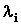scatter off an electron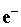at an angle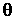like in Fig.1. In collision, it transfers some energy to the electronand emerges at a shift frequency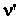or wavelength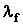as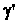.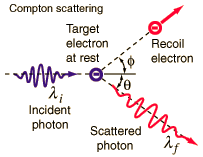Fig.1 Compton effect

Conservation laws give: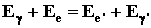(2.1)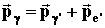or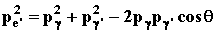(2.2)

where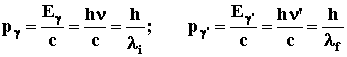(2.3)

and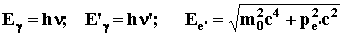(2.4)

Plugging (2.3) and (2.4) into (2.1) and (2.2), one can derive the Compton scattering equation: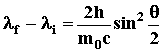where the quantity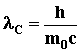is known as the Compton wavelength.